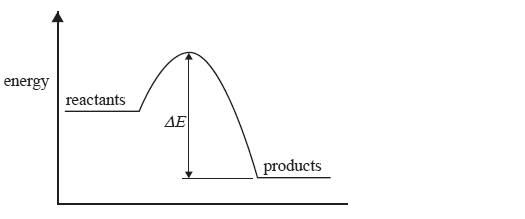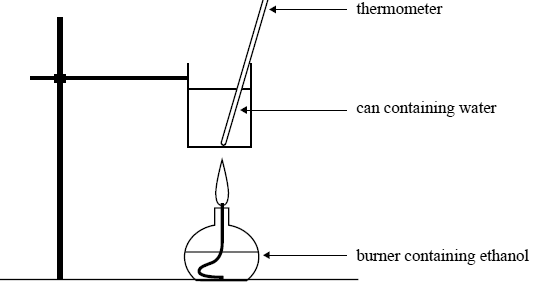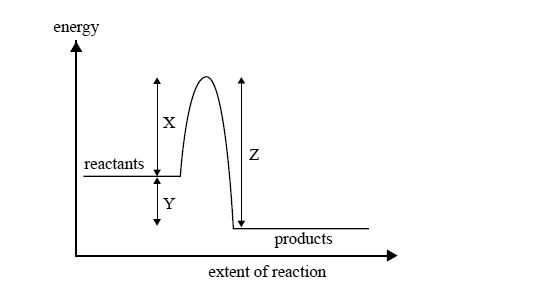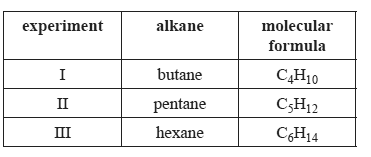Thermochemistry 2009 VCE The change in energy during a reaction is represented in the following energy profile diagram.The change in energy labelled ΔE above is A. the energy absorbed when bonds in the reactants break. B. the activation energy of the forward reaction. C. the activation energy for the reverse reaction. D. the heat of reaction. Solution If, for the reaction C2H5OH(g) + 3O2(g) → 2CO2(g) + 3H2O(l) ; ΔH = −1364 kJ mol–1 then the ΔH value for 4CO2(g) + 6H2O (l) → 2C2H5OH(g) + 6O2(g) would be A. +2728 kJ mol–1 B. +1364 kJ mol–1 C. +682 kJ mol–1 D. –1364 kJ mol–1 SolutionTwo experiments are carried out. Both involve the combustion of 2.09g of ethanol. Experiment 1 Ethanol is used to calibrate a bomb calorimeter. 2.09 g of ethanol is placed in the bomb calorimeter and reacted with excess oxygen. After the reaction is complete, the temperature of the water surrounding the bomb in the calorimeter has increased by 33.2°C. Calculate, to an appropriate number of signifi cant figures, the calibration factor of the calorimeter, in kJ°C–1. Solution The same mass of ethanol is burnt to heat 200 g of water in a can as shown in the following diagram.Initial temperature of water in the can: 25.3°C Mass of water in the can: 200 g Mass of ethanol burnt: 2.09 g Calculate the final temperature of the water in the can. Assume that 60% of the heat from the burning ethanol is transferred to the water. SolutionSince the start of the industrial age, most of the energy used by humans has come from the burning of coal and oil. In that time the amount of CO2 in the air has increased from approximately 0.42% by mass to 0.58% by mass. Assume that the total mass of the earth’s atmosphere is 5.15 × 1018 kg. Calculate the additional mass of CO2, in kg, that has been added to the earth’s atmosphere since the start of the industrial age. Solution If half of this additional CO2 has come from the burning of coal, calculate the total amount of energy, in kJ, that has been produced by burning all this coal, given that C(s) + O2(g) → CO2(g); ΔH = –394 kJ mol–1 For the purposes of this calculation, assume that coal is pure carbon. SolutionThe following reaction can occur to completion in aqueous solution. CH3Cl(aq) + OH–(aq) → CH3OH(aq) + Cl–(aq) The energy change during this process is illustrated byA reaction can occur between a CH3Cl molecule and a hydroxide ion A. every time they collide. B. only when they collide with exactly the energy X. C. only when they collide with an energy equal to Y–Z. D. only when they collide with an energy greater than or equal to energy X. Solution A catalyst appropriate for this reaction will affect the value of A. X only. B. Y only. C. X and Z only. D. X, Y and Z. SolutionMethyl palmitate, C17H34O2, is a component of one type of biochemical fuel. It is a liquid at room temperature. The molar enthalpy of combustion of methyl palmitate was determined using a bomb calorimeter. The calorimeter was calibrated by passing a current of 4.40 amperes at a potential difference of 5.61 volts through an electric heater for 240 seconds. The temperature of the calorimeter rose by 1.75°C. Calculate the calibration factor of the calorimeter. Include the units of the calibration factor with your answer. Solution A 0.529 g sample of methyl palmitate was then burned in excess oxygen in the calorimeter and the temperature rose by a further 6.19°C. The molar mass of methyl palmitate is 270 g mol–1. Calculate the amount of energy, in kJ, absorbed by the calorimeter when the sample of methyl palmitate was burned. Solution Calculate the amount of energy released, in kJ, by the combustion of 1.00 mol of methyl palmitate. Solution The balanced equation for the combustion of liquid methyl palmitate in excess oxygen is 2C17H34O2(l) + 49O2(g) → 34CO2(g) + 34H2O(l). Write the value of ΔH for this reaction, in kJ mol–1. SolutionThe following table contains information about three experiments. In each experiment 0.10 mol of an alkane is burned completely and all the energy released is used to heat 1.00 L of water which was initially at 20°C.In which experiment(s) will the water be heated to its boiling temperature? A. III only B. II and III only C. I and II only D. I, II and III SolutionPotassium hydroxide and hydrochloric acid react in aqueous solution according to the following equation. KOH(aq) + HCl(aq) → KCl(aq) + H2O(l) A 50 mL solution containing 0.025 mol of KOH was mixed rapidly in an insulated vessel with a 50 mL solution containing 0.025 mol of HCl. The temperature increased by 3.5°C. Assuming that the specific heat capacity of the solution is the same as that of water, the enthalpy change, ΔH, of this reaction, in kJ mol−1, is closest to A. –29 B. –59 C. –2.9 × 104 D. –5.9 × 104 Solution